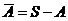# Statistics Assignment Help With Sets And Elements Of Sets

## 2.3 Sets And Elements Of Sets

A set is defined as the collection or aggregate of all possible objects. The objects comprising a set are called elements or points of the set.

### 2.3.1 Operation on sets:

The union of two given sets A and B, denoted by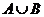, is defined as a set consisting of all possible points which belong to either A or B or both. Thus symbolically,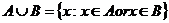The intersection of two sets A and B, denoted by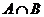, is defined as a set consisting of all those elements which belong to both A and B, thus symbolically,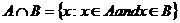If A and B have no common point, that is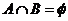, then the sets A and B are said to be disjoint, mutually exclusive or non – overlapping.

The relative difference of a set A from another set B, denoted by A – B is defined as a set consisting of those elements of A which do not belong to B. symbolically,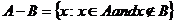The complement or negative of any set A, denoted by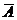is a set containing all elements of the universal set S, that are not elements of A, that is